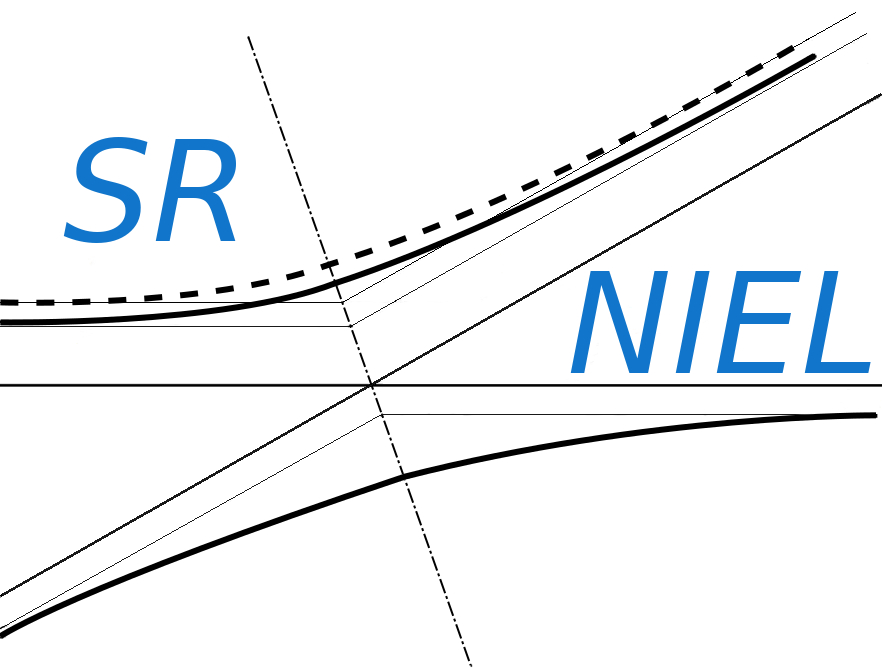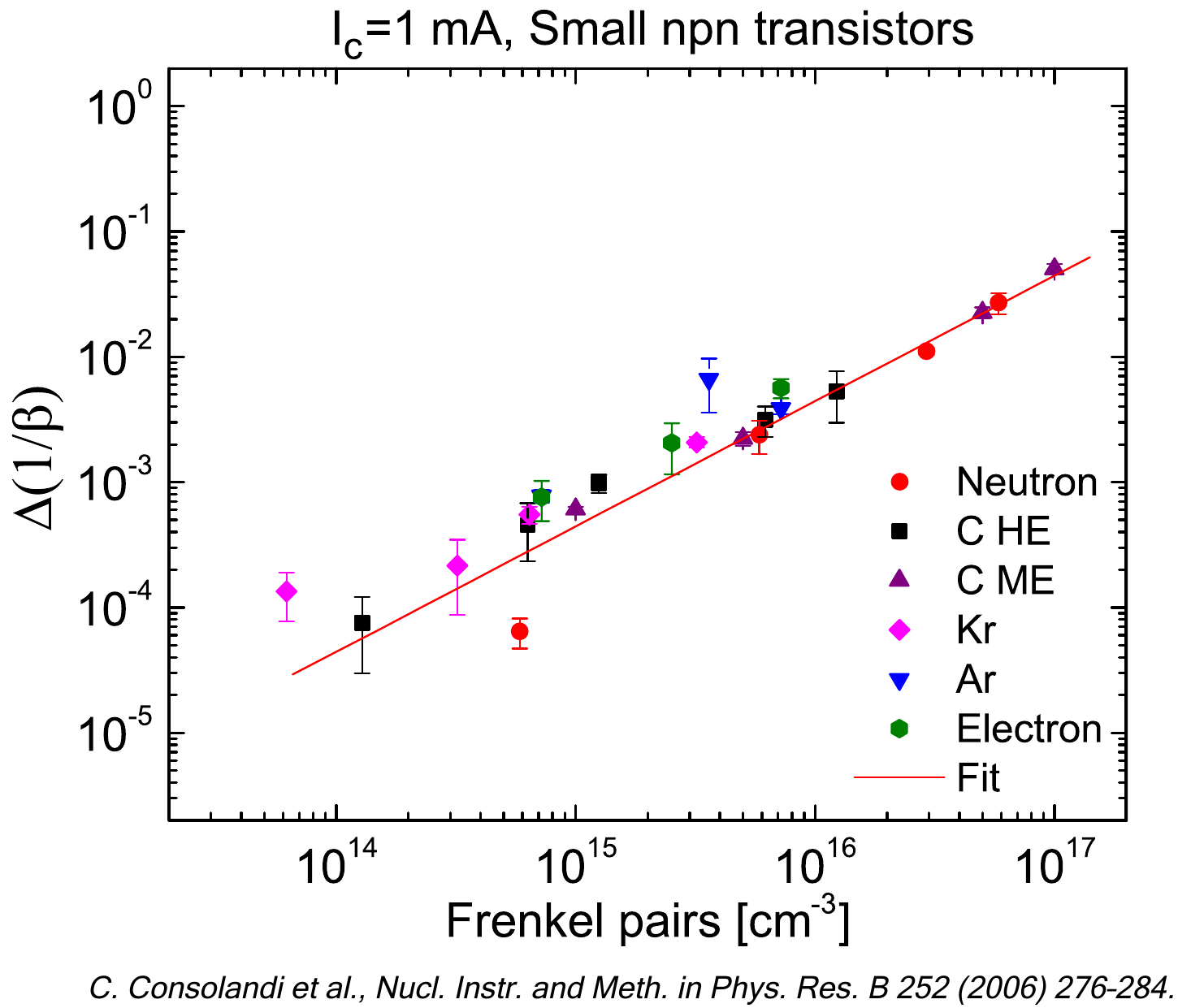SR-NIEL – 7 Screened Relativistic (SR) Treatment for NIEL Dose Nuclear and Electronic Stopping Power Calculator (version 8.0)This is the SR-NIEL Dose calculator. Unregistered users can freely access NIEL Dose calculators based on Robinson partition function.

### How to use this NIEL Calculator

This tool calculates the NIEL Dose curve for a particle incident on a material and for a given spectral fluence. For information about the computation method of NIEL take a look at the NIEL and Displacement Stopping Power page.

The input parameters and options for the tool are described below. When the input form has been completed, pressing the "CALCULATE" button will start the calculation and open the "Results" page (allow for pop-up in your browser settings). The result page will be also linked at the bottom of the calculator page.

### Input Parameters:

- Incident particle (for Protons&Ions Calculator)
- Proton and ion model (for Protons&Ions Calculator)
- Electron form (for Electrons Calculator)
- Target material
- Particle spectral fluence

### Incident Particle

In the Protons&Ions Calculator, using the pull down menu, the user can select the species of the incident particle, either a proton or one of the elemental ions.

### Electron Form Factor

In the Electron Calculator, the user can select the nuclear form factor (Exponential, Gaussian or Uniform ) model to be used. The form factor accounts for the spatial distribution of charge density probed in the electron-nucleus scattering.

### Proton and Ion Model

When a proton or ion is selected as incident particle, it is possible to specify the different contribution to the total non-ionizing energy loss that is returned: the Coulomb scattering contribution, the contribution of the Hadronic interaction or the Total contribution (Hadronic + Coulomb).

In the present NIEL calculators, for protons up to 1 GeV and alpha-particles up to 1 GeV/nucleon impinging on elements with atomic number from Z=4 up to Z=51, as already mentioned in NIEL and Displacement Stopping Power page, the hadronic contributions were interpolated or extrapolated from those obtained from [Jun et al. (20032004,2017)] regarding 10 absobers, i.e., C, Al, Si, P, Ga, Ge, As, In, Cu, Se with corresponding displacement thresold energy of 35, 20, 21, 9, 10, 20, 10, 7, 25, 20 eV, respectively .For silicon absorbers, in addition, the hadronic contribution for energies from 1 up to 24 GeV was obtatined from [Huhtinen (2002)].
As default option (no scaling), no Ed dependence is included for the hadronic contribution to NIEL. It has to be remarked that such a beviour is consistent with the results obtained for neutron damage functions with MeV neutrons (up to 20 MeV) by means of NJOY-2012 code (e.g. see NJOY based neutron NIEL calculator). As alternative option (linearly scaled), the hadronic contribution are linearly varied with respect to the calculated by the same amount found for Coulomb contributions.

### Target Material

In this section it is possible to specify the composition of the target material selecting the number of elements in the compound. The required parameters for each element are:

- Atomic number (Z)/Chemical symbol
- Stoichiometric index or element fraction
- The displacement threshold energy [eV]

### Particle Spectral Fluence

This section define the points of the spectral fluence as a function of energy.
The input format is one point per line (Energy - Flux , separated by a space or tab); it is also possible to copy and paste values.

if Hadronic calculation is included (Hadron only and Coulomb+Hadron cases) the Maximum energy is limited to 1GeV (due to hadronic interaction data availability).

### Result

The result page contains the input parameters, the input spectral fluence and the NIEL curve calculated at the same energies defined in the spectral fluence. For every energy points the spectral NIEL dose is calculated and displayed in the second graph. The spectral NIEL dose is integrated, at the energy steps defined in the spectral fluence, to give the Total NIEL Dose. The result table includes the Spectral fluence, NIEL and spectral NIEL Dose (in [g-1] and [Gy MeV-1]).